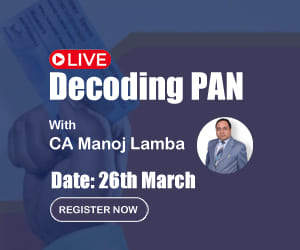# Interest Rate Parity in Foreign Exchange

ESHA AGRAWAL , 29 January 2014

INTEREST RATE PARITY UNDER FOREIGN EXCHANGE

There are large numbers of factors for exchange rate determination but the most important of them are Interest Rate and inflation. Interest Rate parity is a relationship between exchange rate and interest rate.

Let us first discuss the meaning of Interest Rate Parity:- the forward premium or discount on a currency should be such that interest rate across the globe on a covered basis must be equal .This means that whichever country has a lower interest its currency must be at a forward premium and vice versa.

For the sake of precision-

If i\$=9% (interest rate in US 9%)

rupees=4%(interest rate in India 4%)

- dollar should be a forward premium against rupee approximately by 5% and exactly by 5/1.04=4.8%

- rupee should be at a discount against dollar approximately by 5% and exactly by 5/1.09=4.59%

from the meaning of IRP, we conclude that forward premium or discount

=  difference of interest rate factor÷interest rate factor

This can be explained with the help of an example

Let us assume,

interest rates in money market                    exchange rates in forex market

iINR=9%                                                   forward rate INR/\$= 62.88

i\$=4%                                                      spot rate  INR/\$=60

If you look at money market you feel like borrowing in \$ and investing in INR , however in order to make arbitrage profit  you have to pass through forex market twice

Step 1- borrow \$ 1000 @4%. Outflow after 1 year 1000*1.04=1040

Step 2- convert \$ 1000 into spot getting 1000*60= 60000

Step 3- invest INR 60000 at 9% so rupee receivable after 1 yr= 60000*1.09=INR 65400

Step 4- cover yourself , sell INR 65400 one yr forward at forward rate getting 65400/62.88=\$1040

This goes to show that forward premium on \$ should be  (F÷S)*100*(12÷N)=(62.88-60/60)*100*12/12=4.8%

Assuming that the quotation is A/B

(F-S)÷S=(iA-iB)÷(1+iB)

((F-S)÷S)+1=((iA-iB)÷(1+iB))+1

F÷S=(1+iA)÷(1+iB)

In words F÷S=interest rate factor for A÷interest rate factor for B

From the above example we have F÷S=1+iINR÷1+i\$

=F÷60=1+0.9÷1+0.04

F INR/\$= 62.88

The world would have been so beautiful this way, unfortunately we would be required to find out 1mf, 3mf, 6mf but all interest rates are given p.a. so on the right hand side of the equation we have to use periodic interest rate factors. The manner in which annual interest rates are converted into periodic interest factor depend upon nature of compounding. So if we have 9% p.a. and we have to find out 6 months interest rate factor

Ans 1:- 9/2=4.5% , so interest rate factor 1.045 (compounded semi-annually)

Ans 2:- (1.09)^1/2 =1.0440 (compounded annually)

Ans 3:- e^.o45=1.046(compounded continuously)

LOGIC BEHIND IRP

IRP must hold good to prevent arbitrage. Covered interest arbitrage (CIA) , CIA involves four step I am explaining with the help of below mention example:

Spot rate INR/\$= INR60

6 month forward rate INR/\$=INR62

6 month interest rate INR=14%

Showing the process of CIA by borrowing in \$100000 or INR6000000

Let us first of all use the IRP equation to find out the INR interest rate,

That should have prevailed-

f/s= INR interest factor/\$ interest factor

62/60 = 1+i INR/ 1.02

i. INR =  5.40% for six month therefore10.8% p.a(should be)

since actual INR interest rate is 14% which is higher than 10.8%, we should invest INR by borrowing \$.

Arbitrage process

Step 1: borrow \$ 100000 @ 4% interest p.a therefore 2% for six month so outflow after six month 100000* 1.02 = \$ 102000.

Step 2: convert \$100000 spot getting 100000 *60 = INR 6000000

Step 3: invest INR 6000000 at 14% p.a.  therefore 7% for six months so INR receivable on maturity = 6000000 * 1.07= 6420000

Step 4: cover yourself therefore sell INR 6420000 six month forward , \$ inflow after six month = 6420000/62 = \$ 103548

Arbitrage Profit = 103548-102000 = \$ 1548

Because of step one interest rate in \$ rises

Because of step 2 spot rate today in \$ falls

Because of step 3 interest rate in INR falls

Because of step 4 forward rate in \$ rises

At equilibrium, arbitrage profit would be eliminated & IRP will start holding good

i. free flow of capital

ii. no taxes

iii. no transaction cost

Transaction cost in the forex market refers Bto bid-ask spread . transaction cost in the money market refers to difference between borrowing rate and investment rate.

In real life the above three conditions are not satisfied, there are regulations restricting mobility of capital, also taxes and transaction cost are present. This means IRP equation does not hold good in real life.

Esha Agrawal

ESHA AGRAWAL
(job)
Category Others   Report

2 Likes   62 Shares   21810 Views

### Related Articles### Popular Articles#### Trending Online Classes

GST Practitioner Certificate Course 35th BatchCA Arun Chhajer

Refresher Course on Bank Branch AuditSachin Jain

Excel Interactive Dashboards and Data Analysis Certification CourseCA Deepak Gupta

CCI Articles﻿